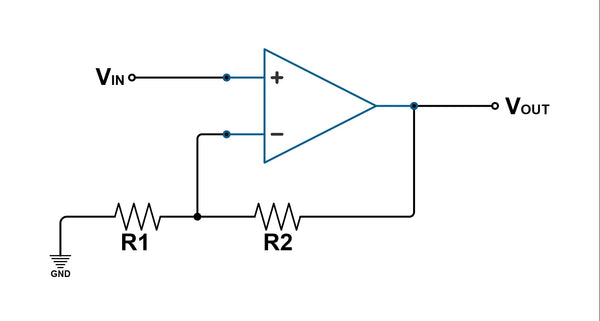Op Amp Gain Calculator - Knowing how to calculate the gain - ShopSolar.com

# Op Amp Gain Calculator

In the world of solar power, terms can be confusing. We use amps in our house amp calculators to see which devices are power-hungry, but what are amps used for in op-amp gain calculators?

Better yet, how does this relate to solar systems?

Join us as we break down everything you need to know about operational amplifier gain and try to link it to solar systems.

## What Are Operational Amplifiers?

Operational amplifiers are used in a variety of analog electronics and solar setups. They take the form of chips as either traditional leaded varieties or circuit mount types.

Their function is to provide gain, where the signal at the input is amplified and appears larger at the output.

The gain is typically measured as voltage gain and is equivalent to the output voltage divided by the input.

Unfortunately, that calculators and methods that convert volts to amps won’t help us out here.

This calculation shows us how much larger the output voltage is compared to the input.

## How They’re Used

When an operational amplifier is being used to provide gain, it has negative feedback applied around it. This feedback provides a defined level of gain, lower distortion, and wider bandwidth.

When a signal is applied to the positive input, it appears at the output in the same phase.

However, if a signal is applied to the negative input, it's inverted as it passed through the output.

This means that the feedback at the negative input is negative feedback that reduces the level of amplification in the circuit.

There are two basic forms of amplifiers that are used. The inverting and non-inverting.

Wrapping your head around inverter amp draw calculators or the op-amp gain calculator can be tricky if you aren't aware of the terminologies.

But now that we’re clued up on op-amp gain, we can look at calculations.

## Inverting Op Amplification

Before we can begin using these chips in solar systems, we should understand how they operate on a smaller scale.

If we have a straight-forward circuit with one resistor from the output to the inverting input, with another from the inverting to the overall circuit, we can apply a signal to the input.

When it reaches the output, the signal becomes inverted, hence the name of this type of amplification.

### CalculationsThe voltage gain for this circuit can be calculated by doing the resistance of the 2nd resistor by the first one.

However, since this is an inverted op amplification, a negative sign must be placed in front of the equation.

Obtaining the voltage in this case may be familiar with using a VA to amps calculator as you’re using values in Ohm’s law.

But let’s slot some values into the resistors. If R1 is 1 Columband R2 is 10 Columb, the gain equation will tell us that the gain is -10.

## Non-Inverting Op Amplification

If we take the same circuit and examine both the input and output signal, we can see that the output would be in the same phase. In other words, it’s not inverted.

### Calculations

The voltage gain equation for this amplifier is different when compared to the inverting op amplification.

It’s still going to be R2 divided by R1, but instead of a negative sign next to it, we’re going to be adding the value of 1.

So, if we use the same values, we’ll end up with a voltage gain of 11, and if we want to be more exact, we’d say plus 11.

## How Can This be Used in Solar Systems?

If you’re wanting to build your solar system, then you could incorporate op-amp chips into circuits.

The combination of resistors, solar panels, a battery like the LiFePo4 12v, and op-amp ICs can make for an effective solar tracker system.If you’re an RV user and you are gathering data from your RV amp hour calculator, you can use this newly acquired knowledge on op-amp gain to control the gain of certain loads.

## Conclusion

To tie everything up into a neat little bow, the negative feedback controls the overall amplifier gain. The inverting amplifier gives us a negative voltage gain, whilst the non-inverting returns a positive.

It’s a useful piece of hardware, as well as a concept and idea, that can be incorporated into our solar systems so that gain can be controlled.

It doesn’t stop there though, we’ve got plenty more resources to help you out. Check out our Ah to kWh calculator to learn more.

Did You Find Our Blog Helpful? Then Consider Checking: